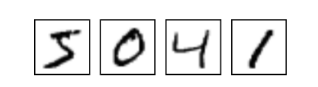02：一文全解：利用谷歌深度学习框架Tensorflow识别手写数字图片（初学者篇）

1.案例背景

MNIST是一个简单的计算机视觉数据集，它是由一系列手写数字图片组成的，比如：2.数据的获取：The MNIST Data

MNIST数据集可以在网站http://yann.lecun.com/exdb/mnist/下载到。 但是在TensorFlow中为了方便学习者获取这个数据，封装了一个方法，我们只需要调用这个方法，程序就会自动去下载和获取数据集。代码如下：

// 导入input_data这个类
from tensorflow.examples.tutorials.mnist import input_data

3.建立多分类模型：Softmax Regressions

3.1 Softmax Regressions原理

Softmax Regressions是一个非常经典的用于多分类模型的方法。就算是之后的笔记中讲到的更复杂的模型，他们的最后一层也是会调用Softmax 这个方法的。

2.2 Tensorflow实现softmax regression

1.要使用tensorflow,实现导入tensorflow的库：

import tensorflow as tf1

2.为输入数据x创建占位符

x = tf.placeholder(tf.float32, [None, 784])1

3.创建两个权重变量 w和b是在训练过程中不断改变不断优化的，使用Variable来创建：

W = tf.Variable(tf.zeros([784, 10]))
b = tf.Variable(tf.zeros())12

4.建立softmax模型

y = tf.nn.softmax(tf.matmul(x, W) + b)1

4.模型训练

1.建立损失函数 上面我们搭建了一个初始的softmax的模型，注意模型中的参数w,b是自己随便定义的。那么训练模型的目的是让模型在学习样本的过程中不断地优化参数，使得模型的表现最好。

y表示模型预测出来的概率分布，y’表示真实的类别概率分布（就是之间one-hot编码之后的标签）。yi表示预测为第i个类的概率， yi’表示真实属于i类的概率（只有i类为1，其余为0）

// 为真实的标签添加占位符
y_ = tf.placeholder(tf.float32, [None, 10])

// 创建交叉熵函数
cross_entropy = tf.reduce_mean(-tf.reduce_sum(y_ * tf.log(y), reduction_indices=))12345

2.使用BP算法优化参数 损失函数就是我们的目标函数，要找到目标函数的最小值，就是对参数求偏导等于0.我们可以使用优化器去不断地降低损失寻找最优参数。比如说最常用的是梯度下降法。代码如下：

3.运行迭代 模型训练的graph基本已经完成，现在我们可以初始化变量，创建会话，来进行循环训练了。

// 创建会话
sess = tf.InteractiveSession()

// 初始化所有变量
tf.global_variables_initializer().run()

//循环1000次训练模型
for _ in range(1000):
# 获取训练集与标签集，每次获取100个样本
batch_xs, batch_ys = mnist.train.next_batch(100)
# 喂数据，训练
sess.run(train_step, feed_dict={x: batch_xs, y_: batch_ys})123456789101112

5.模型评估

correct_prediction = tf.equal(tf.argmax(y,1), tf.argmax(y_,1))1

accuracy = tf.reduce_mean(tf.cast(correct_prediction, tf.float32))1

print(sess.run(accuracy, feed_dict={x: mnist.test.images, y_: mnist.test.labels}))1